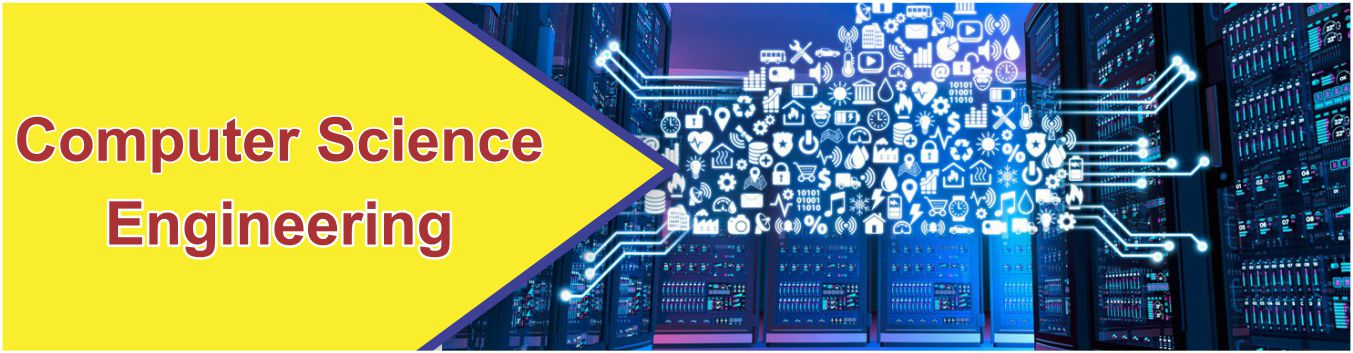# Introduction

## Home IntroductionIntroduction

Computer science is the study of the theory, experimentation, and engineering that form the basis for the design and use of computers. It is the scientific and practical approach to computation and its applications and the systematic study of the feasibility, structure, expression, and mechanization of the methodical procedures (or algorithms) that underlie the acquisition, representation, processing, storage, communication of, and access to information. An alternate, more succinct definition of computer science is the study of automating algorithmic processes that scale. A computer scientist specializes in the theory of computation and the design of computational systems.

Its fields can be divided into a variety of theoretical and practical disciplines. Some fields, such as computational complexity theory(which explores the fundamental properties of computational and intractable problems), are highly abstract, while fields such as computer graphics emphasize real-world visual applications. Other fields still focus on challenges in implementing computation. For example, programming language theory considers various approaches to the description of computation, while the study of computer programming itself investigates various aspects of the use of programming language and complex systems. Human–computer interaction considers the challenges in making computers and computations useful, usable, and universally accessible to humans.

Computer Science & Engineering